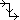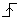# Glossary of Generic Digital Devices

## D Flip-Flop

The digital D-type flip-flop is a one-bit, edge-triggered storage element which stores data whenever the clock (CLK) input line transitions from 0 (low) to 1 (high). In addition, there are asynchronous set and reset signals, which are independent of the clock. When SET = RESET = 0, the data on the D line is transferred to the output Q on the rising edge of the clock. The combination SET = 1 and RESET = 0, causes Q = 1. The combination SET = 0 and RESET = 1 causes Q = 0. The combination SET = RESET = 1 is illegal and is resolved by setting both outputs Q and Q_bar to 1.

Truth Table:

CLK D Q NotesX Qprev Hold State0 0 Data Transfer1 1 Data Transfer

## D Latch

The digital D-type latch is a one-bit, level-sensitive storage element which outputs the value on the data (D) line whenever the enable (EN) input line is 1 (high). The value on the data line is stored, i.e., held on the output (Q) line whenever the enable (EN) line is 0 (low). In addition, there are set and reset signals, which are independent of the enable line. When SET = RESET = 0, the data on the D line is transferred to the output Q whenever EN = 1. The combination SET = 1 and RESET = 0, causes Q = 1. The combination SET = 0 and RESET = 1 causes Q = 0. The combination SET = RESET = 1 is illegal and is resolved by setting both outputs Q and Q_bar to 1.

Truth Table:

EN D Q Notes
0 X Qprev Hold State
1 0 0 Reset
1 1 1 Set

## Digital Buffer

The digital buffer is a single-input, single-output digital device which produces as output a time-delayed copy of its input. The delays associated with the output rise and fall may be different. The model also posts an input load value (in Farads). The output of this model does NOT, however, respond to the total loading it sees on its output; it will always drive the output strongly with the specified delays.

## Digital Clock

The digital clock provides a periodic pulsing input for many other digital devices. Its parameters include the period and pulse width, both expressed in seconds. The pulse width is the time interval during which the clock's output signal is at its logic high level.

## Digital Frequency Divider Block

The digital frequency divider is a programmable step-down divider which accepts an arbitrary divisor (div_factor), a duty cycle term (high_cycles), and an initial count value (i_count). The generated output is synchronized to the rising edges of the input signal. Rise delay and fall delay on the outputs may also be specified independently.

Parameters:

NAME PARAMETER UNIT DEFAULT NOTES
div_factor divide factor - 2 required
high_cycles number of high clock cycles - 1
i_count output initial count value - 0
rise_delay L-to-H delay time sec 1p
fall_delay H-to-L delay time sec 1p

## Digital Input

The digital input provides the easiest way of defining input data in RF.Spice A/D. The data is 1-bit and in decimal format by default. You can define multi-bit data as well as a hexadecimal format. The value of the input can be entered either in the device's property dialog or directly in the Schematic Editor using the up and down arrows of the device symbol.

## Digital Oscillator

The digital oscillator is a mixed-mode device which accepts as input a analog voltage signal. This input is compared to the voltage-to-frequency transfer characteristic specified by the (cntl_array, freq_array) coordinate pairs, and a frequency is obtained which represents a linear interpolation or extrapolation based on those pairs. A digital time-varying signal is then produced with this fundamental frequency. The output waveform, which is the equivalent of a digital clock signal, has rise and fall delays which can be specified independently. In addition, the duty cycle and the phase of the waveform are also variable and can be set by you.

Example SPICE Usage: a5 1 8 var_clock .model var_clock d_osc cntl_array = [-2 -1 1 2] freq_array = [1e3 1e3 10e3 10e3] duty_cycle = 0.4 init_phase = 180.0 rise_delay = 10e-9 fall_delay=8e-9)

Parameters:

NAME PARAMETER UNIT DEFAULT NOTES
cntl_array control array V [0.0] required
freq_array frequency array Hz [1u] required
duty_cycle output duty cycle - 0.5
init_phase intial phase of output deg 0
rise_delay rise delay time sec 1n
fall_delay fall delay time sec 1n

## Digital Output

The digital output provides the easiest way of displaying output data in RF.Spice A/D. The data is in decimal format by default. You can display it in the hexadecimal format.

## Digital Probe

The digital probe is very similar to digital output but does not display the value of the data. It is intended as an output signal designator for transient tests.

## Digital Source

The digital source provides for straightforward descriptions of digital signal vectors in a tabular format. The device reads input from a table or an input file and, at the times specified, and generates the inputs along with the strengths listed. The data is 1-bit and in decimal format by default. You can define multi-bit data as well as a hexadecimal format. You can also make the data periodic with a specified period in seconds or define an initial delay in seconds.

The format of the input file is as shown below:

∗ time value

0 0

10n 1

20n 1

30n 0

40n 1

50n 0

## JK Flip-Flop

The digital JK-type flip-flop is a one-bit, edge-triggered storage element which stores data whenever the clock (CLK) input line transitions from 0 (low) to 1 (high). If J = 1 and K = 0, then the output is set (i.e. Q = 1) on the rising edge of the clock. If J = 0 and K = 1, then the output is reset (i.e. Q = 0). If J = K = 0, then the outputs do not change. If J = K = 1, then the outputs toggle on the positive edge of the clock signal. In addition, there are asynchronous set and reset signals, which are independent of the clock. The combination SET = 1 and RESET = 0, causes Q = 1. The combination SET = 0 and RESET = 1 causes Q = 0.

Truth Table:

CLK J K Q NotesX X Qprev Hold State0 0 Qprev Hold State0 1 0 Reset1 0 1 Set1 1 NOT(Qprev) Toggle

## Logic AND Gate

The AND gate performs the logical function Y = A & B. The AND function's output is one (high) if all of the inputs are one, and zero (low) otherwise.

A B A .AND. B
0 0 0
0 1 0
1 0 0
1 1 1

## Logic Inverter Gate

The Inverter performs the logical function Y = NOT(A). The Inverter function's output is one (high) if the input is zero (low), and vice versa.

A .NOT. A
0 1
1 0

## Logic NAND Gate

The NAND gate is just the inverse of the AND function: Y = NOT(A & B) = NOT(A) | NOT(B). The NAND function's output is zero (low) if all of the inputs are one (high), and one otherwise.

A B A .NAND. B
0 0 1
0 1 1
1 0 1
1 1 0

## Logic NOR Gate

The NOR gate is just the inverse of the OR function: Y = NOT(A | B) = NOT(A) & NOT(B). The NOR function's output is zero (low) if one or more of the inputs are one (high), and one otherwise.

A B A .NOR. B
0 0 1
0 1 0
1 0 0
1 1 0

## Logic OR Gate

The OR gate performs the logical function Y = A | B. The OR function's output is one (high) if one or more inputs are one, and zero (low) otherwise.

A B A .OR. B
0 0 0
0 1 1
1 0 1
1 1 1

## Logic XOR Gate

The XOR gate performs the logical Exclusive OR function Y = A ⊕ B. The XOR function's output is one (high) if one and only one input is one, and zero (low) otherwise.

A B A .XOR. B
0 0 0
0 1 1
1 0 1
1 1 0

## Logic XNOR Gate

The OR gate performs the logical function Y = NOT(A ⊕ B). The XNOR function's output is one (high) if and only if all of the inputs have the same state, and zero (low) otherwise.

A B A .XNOR. B
0 0 1
0 1 0
1 0 0
1 1 1

## Pseudo-Random Bit Sequence Generator

This device outputs a random binary bit at each clock cycle.

Parameters:

None

## SR Flip-Flop

The digital SR-type flip-flop is a one-bit, edge-triggered storage element which stores data whenever the clock (CLK) input line transitions from 0 (low) to 1 (high). The value stored on the output Q line will depend on the S and R input line values. If S = 1 and R = 0, the output is set (i.e. Q = 1) on the rising edge of the clock. If S = 0 and R = 1, the output is reset (i.e. Q = 0) on the rising edge of the clock. If both inputs are S = R = 0, then the outputs do not change from the previous state. If both inputs are S = R = 1, then the result is unknown. In addition, there are asynchronous set and reset signals, which are independent of the clock input. The combination SET = 1 and RESET = 0, causes Q = 1. The combination SET = 0 and RESET = 1 causes Q = 0.

Truth Table:

CLK S R Q NotesX X Qprev Hold State0 0 Qprev Hold State0 1 0 Reset1 0 1 Set1 1 X Illegal

## SR Latch

The digital SR-type latch is a one-bit, level-sensitive storage element which outputs the value dictated by the state of the S and R input lines whenever the enable (EN) input line is 1 (high). This value is stored (i.e., held on the output line) whenever the enable (EN) line is 0 (low). If S = 1 and R = 0, the latch is set (i.e. Q = 1). If S = 0 and R = 1, the latch is reset (i.e. Q = 0). If both inputs are S = R = 0, then the outputs do not change from the previous state. If both inputs are S = R = 1, then the result is unknown. In addition, there are set and reset signals, which are independent of the enable line. The combination SET = 1 and RESET = 0, causes Q = 1. The combination SET = 0 and RESET = 1 causes Q = 0.

Truth Table:

EN S R Q Notes
0 X X Qprev Hold State
1 0 0 Qprev Hold State
1 0 1 0 Reset
1 1 0 1 Set
1 1 1 X Illegal

## Toggle Flip-Flop

The digital T-type flip-flop is a one-bit, edge-triggered storage element which toggles its current state whenever the clock (CLK) input line transitions from 0 (low) to 1 (high). When the toggle (T) line is zero, the flip-flop is inactive. When T is high, the flip-flop's output toggles its value on the rising edge of the clock. In addition, there are asynchronous set and reset signals, which are independent of the clock input. The combination SET = 1 and RESET = 0, causes Q = 1. The combination SET = 0 and RESET = 1 causes Q = 0.

Truth Table:

T CLK Q Notes
0 X Qprev Hold State
1Qprev Hold State
1NOT(Qprev) Toggle